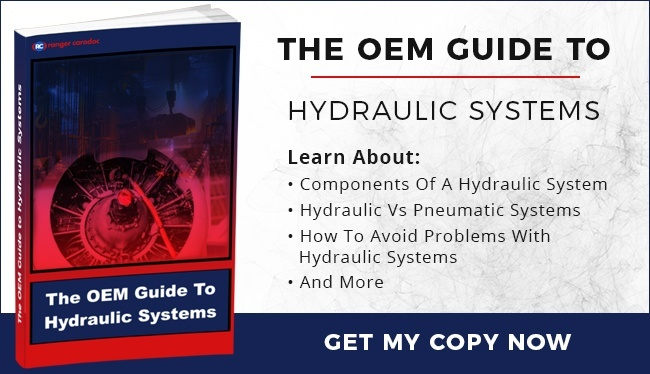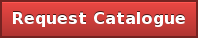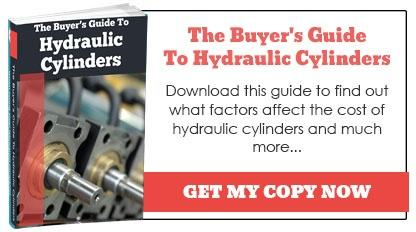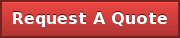# Ranger Caradoc Hydraulics Ltd BlogOrdering the wrong type of hydraulic cylinder can work out expensive. This is easier to do than it might appear, as many product numbers are similar and some suppliers are unwilling or unable to provide the bespoke advice needed to place the correct order.

Our advice team are all experienced hydraulic engineers and can help you get the right size first time. In the meantime, this quick guide will help you avoid common mistakes and make the order process easier.

## Why Size Matters

The size, in terms of bore and surface area of a hydraulic cylinder, directly affects the available force and internal pressure – thus determining its potential power output. It therefore makes sense to work backwards from the capacity required from your cylinder before settling on size.

The risk of miscalculating the correct size is a cylinder with insufficient strength or not enough speed to carry out its function.

## Calculating Area & Force

The most important measurement when determining size is the amount of pressure on the piston, as this determines the cylinder’s lifting capacity, or force. Pressure is measured in psi, or pounds per square inch, so the size we are most concerned with is the area (in square inches) at the bottom of the piston.

How is this determined?

If you already know the bore size of your cylinder (e.g. if you are using ISO standard hydraulic cylinders) then you can determine piston area size using the following formula:

Mathematically, the area of a circular piston can be calculated by, where A is area and r is the radius. From an engineering perspective, however, it is difficult to calculate the radius directly. We therefore take the diameter (D) and divide it by two.

This gives the equation 2, or , as both the ‘2’ and the diameter are squared.

For an 80 mm / 3.14 inch bore, you would have the following piston area:

A = 7.7 square inches at the piston base.

To calculate maximum possible lift you need to know the pressure input from your hydraulic pump in psi. If this was 1000 psi, for instance, the maximum lift of your hydraulic cylinder would be 7700 lb.

This load isn’t normally achievable in practice, however, because of counteracting pressure from the upper side of the piston. This can happen for several reasons, such as the piping leading to the rod end being too small. Allowance must be made for this when determining bore size, piston area and lift capacity.

## Calculating Bore

If you know the pressure input and force output you need but not the cylinder diameter (bore), you can find the right size by using the following equation:

Force divided by pressure will yield the area at the piston base. So...

If the force is 4000 lbs and the pressure is 1000 psi, the area will be four square inches.

By running the equation above you reach a diameter/bore of 2.25 inches, or 58 mm.

## Support Ordering The Right Parts

Ordering the wrong size of hydraulic cylinder can have negative ramifications for your application. Get it right first time with our personalised product ordering advice service. As a well-established manufacturer and supplier of hydraulic cylinders, we can ensure you get the right specs for your project each time. Call 01299 896953 for more details.Topics: Hydraulic Cylinders

### Subscribe Here!